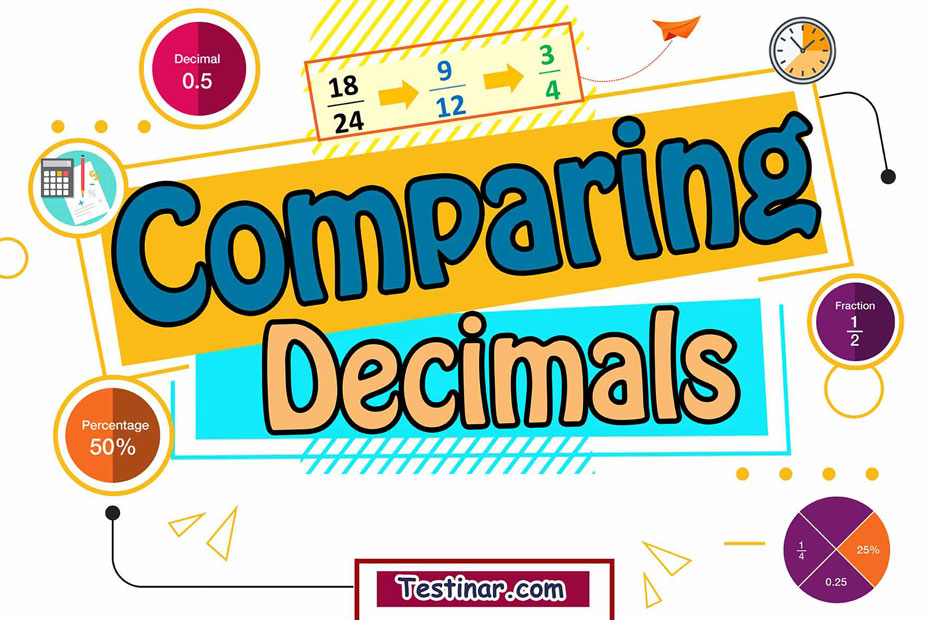## How to Compare Decimals

A decimal is a number that consists of a whole number and a fractional part. To put it in another way, decimals are fractions and mixed numbers with denominators that are powers of 10. For example, the decimal  $$0.6$$  is the same as the fraction  $${ 6 \over 10}$$  , or the decimal  $$1.2$$  is the same as fraction  $${12 \over 10}$$  or the mixed number  $$1{1 \over 5}$$ .  You can change Fractions and mixed numbers to decimals. For instance, instead of writing  $$4 \over 5$$  you can write  $$0.8$$  . Below are few steps for comparing two decimals:

Steps To compare two decimals:
Step 1:
Compare the whole parts first. if the whole part of one decimal is greater, it means that this decimal is greater than the other.
Step 2: But if the whole parts are the same, compare the decimals from the left. (hundreds, tens, ones, tenth, hundredth, etc.)
Step 3: To compare decimals, use the following symbols:
Equal to $$=$$
Less than$$\lt$$
Greater than $$\gt$$
Greater than or equal $$\geq$$
Less than or equal $$\leq$$

Example:
$$2.67 \gt 0.267$$

### Exercises for Comparing Decimals

1) $$43.97 \ \bbox[5px,border:2px solid black] { } \ 2.83$$

2) $$58.75 \ \bbox[5px,border:2px solid black] { } \ 55.24$$

3) $$65.5 \ \bbox[5px,border:2px solid black] { } \ 42.81$$

4) $$6.18 \ \bbox[5px,border:2px solid black] { } \ 6.18$$

5) $$199.5 \ \bbox[5px,border:2px solid black] { } \ 19.95$$

6) $$462.7 \ \bbox[5px,border:2px solid black] { } \ 46.27$$

7) $$13.24 \ \bbox[5px,border:2px solid black] { } \ 13.29$$

8) $$789.5 \ \bbox[5px,border:2px solid black] { } \ 78.95$$

9) $$31.43 \ \bbox[5px,border:2px solid black] { } \ 31.62$$

10) $$46.82 \ \bbox[5px,border:2px solid black] { } \ 15.66$$

1)$$43.97 \ \bbox[5px,border:2px solid black] {\color{red}{\gt}} \ 2.83$$
Solution:
In this example, because the whole number of $$43.97$$ is greater than $$2.83$$ , therefore $$43.97 \ \bbox[5px,border:2px solid black] {\color{red}{\gt}} \ 2.83$$
2) $$58.75 \ \bbox[5px,border:2px solid black] {\color{red}{\gt}} \ 55.24$$
Solution:
In this example, because the whole number of $$58.75$$ is greater than $$55.24$$ , therefore $$58.75 \ \bbox[5px,border:2px solid black] {\color{red}{\gt}} \ 55.24$$
3) $$65.5 \ \bbox[5px,border:2px solid black] {\color{red}{\gt}} \ 42.81$$
Solution:
In this example, because the whole number of $$65.5$$ is greater than $$42.81$$ , therefore $$65.5 \ \bbox[5px,border:2px solid black] {\color{red}{\gt}} \ 42.81$$
4) $$6.18 \ \bbox[5px,border:2px solid black] {\color{red}{=}} \ 6.18$$
Solution:
In this example, because the whole numbers and fractional parts are the same. Therefore they are equal: $$6.18 \ \bbox[5px,border:2px solid black] {\color{red}{=}} \ 6.18$$
5) $$199.5 \ \bbox[5px,border:2px solid black] {\color{red}{\gt}} \ 19.95$$
Solution:
In this example, because the whole number of $$199.5$$ is greater than $$19.95$$ , therefore $$199.5 \ \bbox[5px,border:2px solid black] {\color{red}{\gt}} \ 19.95$$
6) $$462.7 \ \bbox[5px,border:2px solid black] {\color{red}{\gt}} \ 46.27$$
Solution:
In this example, because the whole number of $$462.7$$ is greater than $$46.27$$ , therefore $$462.7 \ \bbox[5px,border:2px solid black] {\color{red}{\gt}} \ 46.27$$
7) $$13.24 \ \bbox[5px,border:2px solid black] {\color{red}{\lt}} \ 13.29$$
Solution
In this example, the whole numbers are the same, therefore we compare the fractional parts. Since the fraction part $$0.29$$ is greater than $$0.24$$ , so $$13.24 \ \bbox[5px,border:2px solid black] {\color{red}{\lt}} \ 13.29$$
8) $$789.5 \ \bbox[5px,border:2px solid black] {\color{red}{\gt}} \ 78.95$$
Solution:
In this example, because the whole number of $$789.5$$ is greater than $$78.95$$ , therefore $$789.5 \ \bbox[5px,border:2px solid black] {\color{red}{\gt}} \ 78.95$$
9) $$31.43 \ \bbox[5px,border:2px solid black] {\color{red}{\lt}} \ 31.62$$
Solution
In this example, the whole numbers are the same, therefore we compare the fractional parts. Since the fraction part $$0.62$$ is greater than $$0.43$$ , so $$13.43 \ \bbox[5px,border:2px solid black] {\color{red}{\lt}} \ 13.62$$
10) $$46.82 \ \bbox[5px,border:2px solid black] {\color{red}{\gt}} \ 15.66$$
Solution:
In this example, because the whole number of $$46.82$$ is greater than $$15.66$$ , therefore $$46.82 \ \bbox[5px,border:2px solid black] {\color{red}{\gt}} \ 15.66$$

## Compare Decimals Quiz

### The Most Comprehensive Praxis Core Math Preparation Bundle

$76.99$36.99

### ALEKS Math Full Study Guide

$25.99$12.99

### GED Math Full Study Guide 2022-2023

$25.99$13.99

### ISEE Middle Level Math Comprehensive Prep Bundle

$89.99$47.99